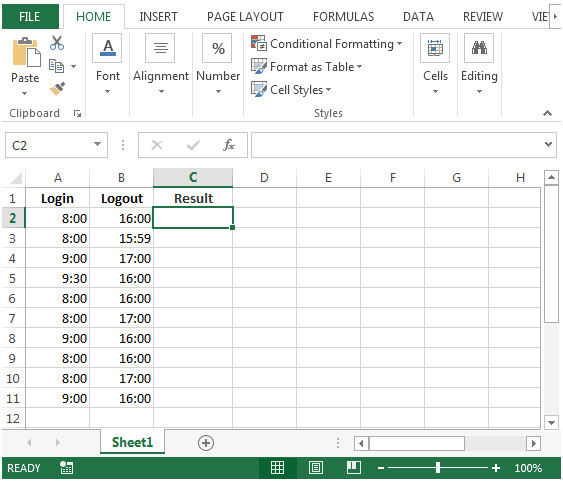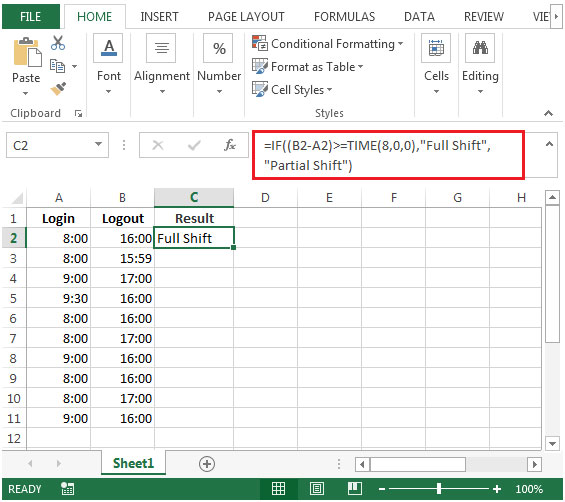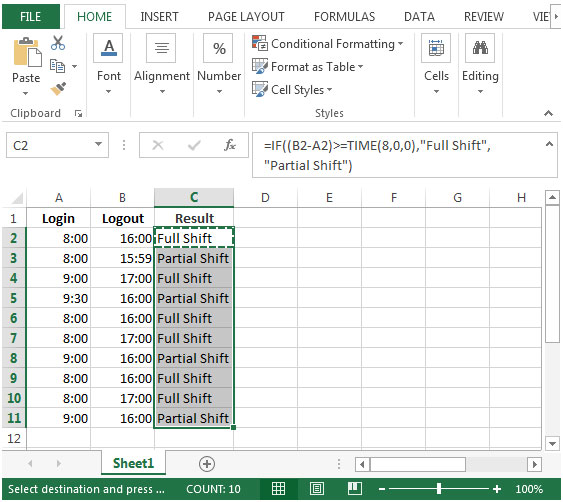# Determining whether a Person Worked in a Full Or Partial Shift in Excel 2010

In this article, we will learn how to determine whether a person worked a full or partial shift.

While preparing attendance report, you want a formula to find out whether a person has worked in a full or partial shift.

We can find the output by using IF function.

The IF function checks if a condition you specify is TRUE or FALSE. If the condition returns TRUE then it returns preset value, and if the condition returns FALSE then it returns another preset value.

Syntax = IF(logical_test,value_if_true,value_if_false)

logical_test: Logical test will test the condition or criteria. If condition meets then it returns the preset value, and if condition does not meet, then it returns another preset value.

value_if_true: The value that you want to be returned if this argument returns TRUE.

value_if_false: The value that you want to be returned if this argument returns FALSE

Let us take an example:

• We have Login & Logout time in column A & B respectively.

We want a formula to derive the output whether a person has worked in a full or partial shift. Partial shift here refers that a person has not completed at least 8 hours in a day.• In cell C2, the formula would be
=IF((B2-A2)>=TIME(8,0,0),"Full Shift","Partial Shift")• The IF formula will check if the difference of Login & Logout Time is greater than 8, if condition found TRUE then the result would “Full Shift” otherwise result would be “Partial Shift”.
• Copying the formula in cell C2 to range C3:C11, we will get desired result.Terms and Conditions of use

The applications/code on this site are distributed as is and without warranties or liability. In no event shall the owner of the copyrights, or the authors of the applications/code be liable for any loss of profit, any problems or any damage resulting from the use or evaluation of the applications/code.